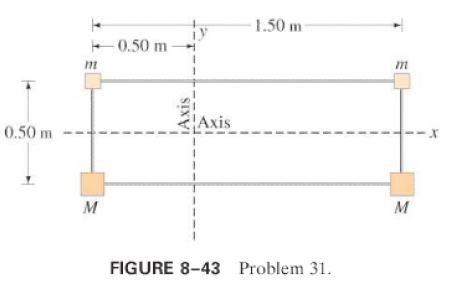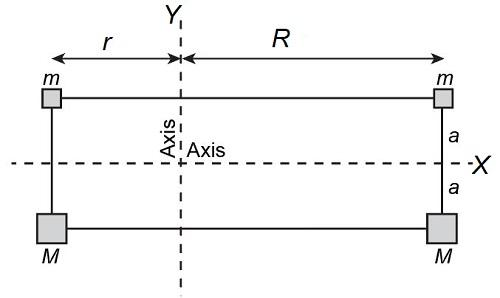×
Get Full Access to Physics: Principles With Applications - 6 Edition - Chapter 8 - Problem 31p
Get Full Access to Physics: Principles With Applications - 6 Edition - Chapter 8 - Problem 31p

×

# Calculate the moment of inertia of the array of pointISBN: 9780130606204 3

## Solution for problem 31P Chapter 8

Physics: Principles with Applications | 6th Edition

• Textbook Solutions
• 2901 Step-by-step solutions solved by professors and subject experts
• Get 24/7 help from StudySoup virtual teaching assistantsPhysics: Principles with Applications | 6th Edition

4 5 1 246 Reviews
11
0
Problem 31P

(II) Calculate the moment of inertia of the array of point objects shown in Fig.aboutthe vertical axis, andthe horizontal axis. Assume., and the objects are wired together by very light, rigid pieces of wire. The array is rectangular and is split through the middle by the horizontal axis. () About which axis would it be harder to accelerate this array?Step-by-Step Solution:

Step 1 of 4

The schematic diagram for the problem can be drawn as:Step 2 of 4

Step 3 of 4

##### ISBN: 9780130606204

The answer to “?(II) Calculate the moment of inertia of the array of point objects shown in Fig. about the vertical axis, and the horizontal axis. Assume . , and the objects are wired together by very light, rigid pieces of wire. The array is rectangular and is split through the middle by the horizontal axis. ( ) About which axis would it be harder to accelerate this array?” is broken down into a number of easy to follow steps, and 66 words. This textbook survival guide was created for the textbook: Physics: Principles with Applications, edition: 6. The full step-by-step solution to problem: 31P from chapter: 8 was answered by , our top Physics solution expert on 03/03/17, 03:53PM. Physics: Principles with Applications was written by and is associated to the ISBN: 9780130606204. Since the solution to 31P from 8 chapter was answered, more than 1404 students have viewed the full step-by-step answer. This full solution covers the following key subjects: axis, array, horizontal, objects, accelerate. This expansive textbook survival guide covers 35 chapters, and 3914 solutions.

## Discover and learn what students are asking

Calculus: Early Transcendental Functions : Inverse Trigonometric Functions: Integration
?In Exercises 1-20, find the indefinite integral. $$\int \frac{1}{x \sqrt{x^{4}-4}} d x$$

Statistics: Informed Decisions Using Data : Hypothesis Tests Regarding a Parameter
?A test is conducted at the = 0.05 level of significance. What is the probability of a Type I error?

Unlock Textbook Solution